#### Common Notation

Table 71.29 displays notation for some of the more common parameters across analyses. The Associated Syntax column shows examples of relevant analysis statement options, where applicable.

Table 71.29: Common Notation

Symbol

Description

Associated SyntaxSignificance level

ALPHA=

N

Total sample size

NTOTAL=, NPAIRS=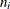Sample size in ith group

NPERGROUP=, GROUPNS=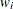Allocation weight for ith group (standardized to sum to 1)

GROUPWEIGHTS=
(Arithmetic) mean

MEAN=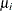(Arithmetic) mean in ith group

GROUPMEANS=, PAIREDMEANS=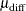(Arithmetic) mean difference,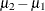or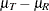MEANDIFF=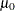Null mean or mean difference (arithmetic)

NULL=, NULLDIFF=
Geometric mean

MEAN=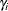Geometric mean in ith group

GROUPMEANS=, PAIREDMEANS=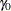Null mean or mean ratio (geometric)

NULL=, NULLRATIO=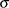Standard deviation (or common standard deviation per group)

STDDEV=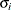Standard deviation in ith group

GROUPSTDDEVS=, PAIREDSTDDEVS=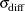Standard deviation of differences

CV

Coefficient of variation, defined as the ratio of the standard deviation to the (arithmetic) mean

CV=, PAIREDCVS=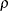Correlation

CORR=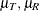Treatment and reference (arithmetic) means for equivalence test

GROUPMEANS=, PAIREDMEANS=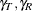Treatment and reference geometric means for equivalence test

GROUPMEANS=, PAIREDMEANS=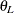Lower equivalence bound

LOWER=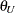Upper equivalence bound

UPPER=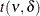t distribution with df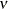and noncentrality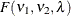F distribution with numerator df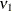, denominator df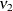, and noncentrality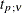pth percentile of t distribution with df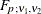pth percentile of F distribution with numerator dfand denominator df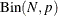Binomial distribution with sample size N and proportion p

A lower one-sided test is associated with SIDES=L (or SIDES=1 with the effect smaller than the null value), and an upper one-sided test is associated with SIDES=U (or SIDES=1 with the effect larger than the null value).

Owen (1965) defines a function, known as Owen’s Q, that is convenient for representing terms in power formulas for confidence intervals and equivalence tests: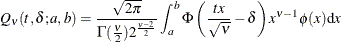where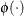and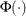are the density and cumulative distribution function of the standard normal distribution, respectively.